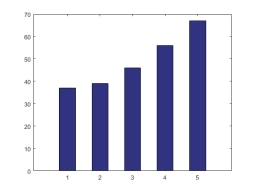# A group 2

A group of students was asked, "How many hours did you watch television last week? " Here are their responses.
13, 14, 4, 19, 19, 11
Find the median and mean number of hours for these students.
(a) Median:

(b) Mean:

m1 =  13.5
m2 =  10.2

### Step-by-step explanation:Did you find an error or inaccuracy? Feel free to write us. Thank you!

Tips for related online calculators
Looking for help with calculating arithmetic mean?
Looking for a statistical calculator?

#### Grade of the word problem:

We encourage you to watch this tutorial video on this math problem: